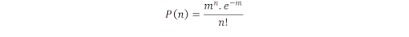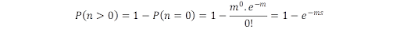8:02 pm

# The multiplicity of infection (MOI) of the virus (bacteriophage) explained

The multiplicity of infection or MOI is the ratio of the infectious agent (e.g. phage or virus) to infection targets (e.g. bacterial cell). For example, when referring to a group of bacterial cells (culture) inoculated with infectious virus particles, the multiplicity of infection or MOI is the ratio defined by the number of infectious virus particles inoculated divided by the number of target cells present in that bacterial culture. This step is usually done after bacteriophage isolation and purification steps due to the fact that crude phage preparation will not produce reliable results.

Interpretation

The actual number of phages or viruses that will enter any given cell is a statistical process: some cells may absorb more than one virus particle while others may not absorb any (it is a matter of assumption and probability). The probability that a cell will absorb n virus particles when inoculated with an MOI of m can be calculated for a given population using a Poisson distribution.where m is the multiplicity of infection or MOI, n is the number of infectious agents that enter the infection target, and P(n) is the probability that an infection target (a cell) will get infected by n infectious agents.

In fact, the infectivity of the virus in question will alter this relationship. One way around this is to use a functional definition of infectious particles rather than a strict count, such as a plaque-forming unit.

For example, when an MOI of 1 (1 viral particle per cell) is used to infect a population of cells, the probability that a cell will not get infected is P(0) = 36.79%, and the probability that it is infected by a single particle is P(1) = 36.79%, by two particles is P(2) = 18.39%, by three particles is P(3) = 6.13%, and so on.

The average percentage of cells that will become infected as a result of inoculation with a given MOI can be obtained by realizing that it is simply P(n >0) = 1 − P(0). Hence, the average fraction of cells that will become infected following inoculation with an MOI of m is given by:which is approximately equal to m for small values of m << 1.

### MOI Examples

Percentage of cells infected based on MOI. As the MOI increases, the percentage of cells infected with at least one viral particle also increases.

MOI % Infected

1.0

63.2%

2.0

86.5%

3.0

95.0%

4.0

98.2%

5.0

99.3%

6.0

99.8%

7.0

99.9%

8.0

100.0%

MOI is one of the important parameters in the phage research world. It is important for one interested in phage work to know how to do this practically
References

Fields’ virology, Part 1 By Bernard N. Fields, David Mahan Knipe, Peter M. Howley, Diane E. Griffin

(Visited 5 times, 1 visits today)